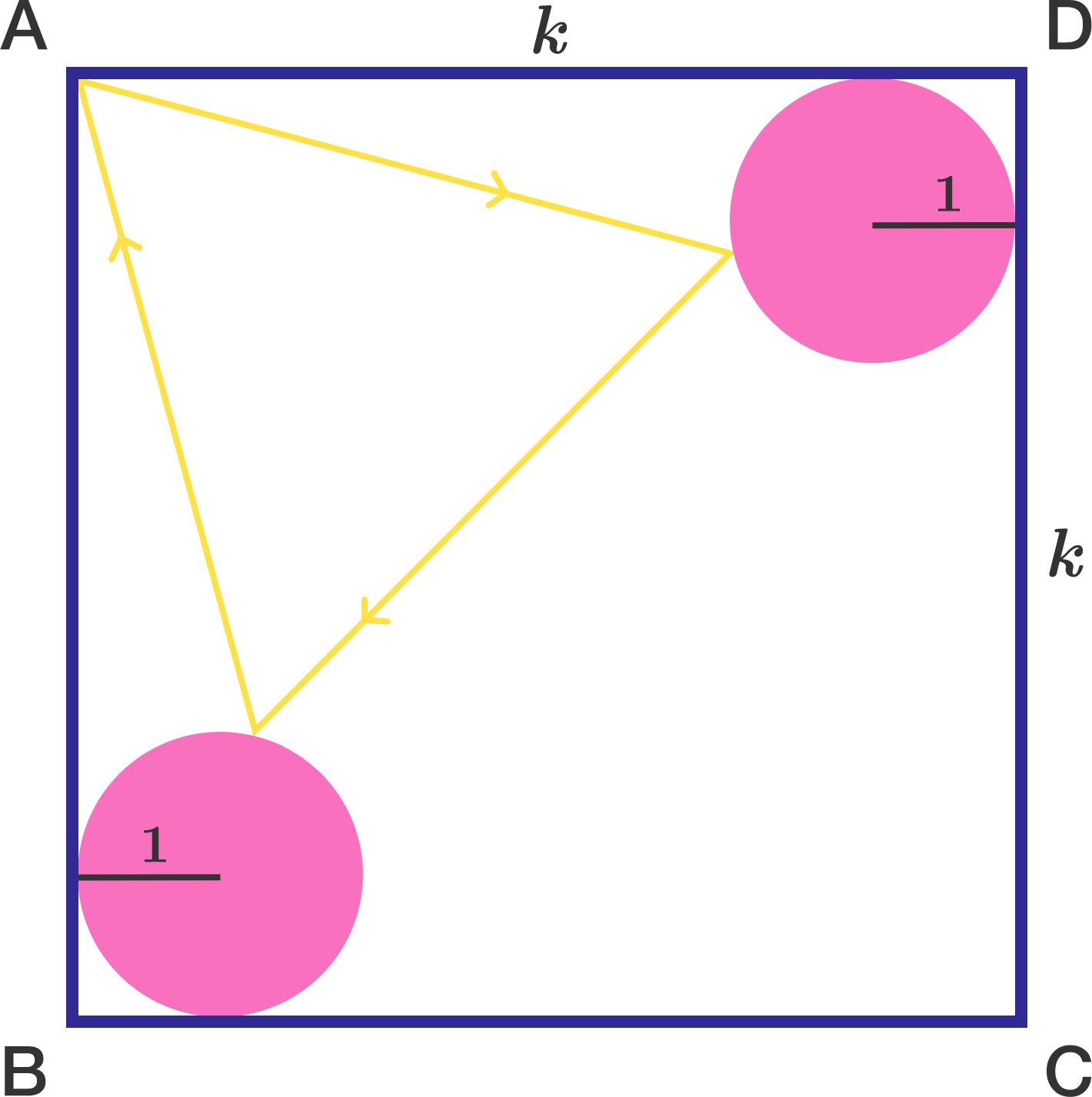# Reflect on this...

Geometry Level 4

A square $ABCD$ of side length $k$ contains unit circles at each of corners $B$ and $D$ such that each circle is tangent to the square at precisely two points. A ray of light emanating from point $A$ reflects off each circle and then returns to $A$, creating a path in the shape of an equilateral triangle.

There is a unique value of $k$ for which this scenario can occur. Find $\lfloor 10000\cdot k \rfloor$.

Note: "Reflecting" means that the angle of incidence equals the angle of reflection.×